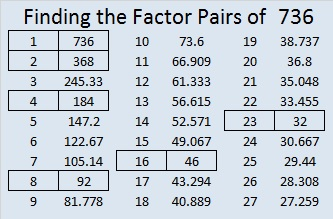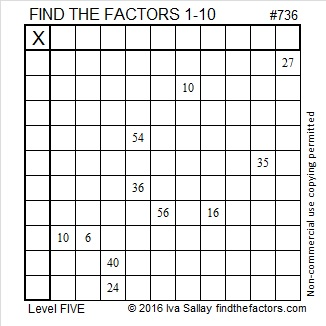# 736 and Level 5

• 736 is a composite number.
• Prime factorization: 736 = 2 x 2 x 2 x 2 x 2 x 23, which can be written 732 = (2^5) x 23
• The exponents in the prime factorization are 5 and 1. Adding one to each and multiplying we get (5 + 1)(1 + 1) = 6 x 2 = 12. Therefore 736 has exactly 12 factors.
• Factors of 736: 1, 2, 4, 8, 16, 23, 32, 46, 92, 184, 368, 736
• Factor pairs: 736 = 1 x 736, 2 x 368, 4 x 184, 8 x 92, 16 x 46, or 23 x 32
• Taking the factor pair with the largest square number factor, we get √736 = (√16)(√46) = 4√46 ≈ 27.1293199.Some great online resources for teachers are on the Mathfireworks website. I am very pleased that Find the Factors made the list. It is included under Puzzles and Games. Help for solving today’s puzzle can be found in the table at the bottom of this post.Print the puzzles or type the solution on this excel file: 10 Factors 2016-01-04

———————————————————

23 ⋅ 32 = 736 so it is the product of semordnilaps. (Semordnilap is palindromes spelled backwards, so semordnilap and palindromes are semordnilaps.)

736 = 7 + 3^6 making it the 14th Friedman number. Since 736 is equal to an expression that uses only “+ – × / ^ ( )”, all of its digits (or a concatenation of its digits) in the order in which they occur in the number, it is the 3rd nice Friedman number. Thank you OEIS.org for that fun number fact. (All of the suggested links are different and well worth a look.) Note: (736) = 736 is trivial so it would not satisfy the definition of a Friedman number.

21 + 22 + 23 + 24 + 25 + 26 + 27 + 28 + 29 + 30 + 31 + 32 + 33 + 34 + 35 + 36 + 37 + 38 + 39 + 40 + 41 + 42 + 43 = 736; that’s 23 consecutive numbers.

735 and 736 are the smallest pair of consecutive numbers with 12 factors each.

736 is a palindrome in 2 bases:

• 1E1 BASE 21 (E = 14 base 10); note that 1(21^2) + 14(21^1) + 1(21^0) = 736.
• NN BASE 31 (N = 23 base 10); note that 23(31) + 23(1) = 736.

———————————————————This site uses Akismet to reduce spam. Learn how your comment data is processed.